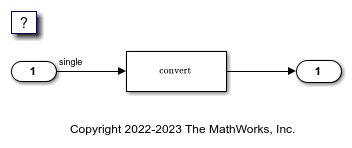# Floating-Point Multiplication to Handle a Net Slope Correction

This example shows how to use floating-point multiplication to handle a net slope correction. When converting floating-point data types to fixed-point data types in the generated code, a net slope correction is one method of scaling fixed-point data types. Scaling the fixed-point data types avoids overflow conditions and minimizes quantization errors.

For processors that support efficient multiplication, using floating-point multiplication to handle a net slope correction improves code efficiency. If the net slope correction has a value that is not a power of two, using division improves precision.

Note: This example requires a Fixed-Point Designer™ license.

### Example

In the model rtwdemo_float_mul_for_net_slope_correction, a Convert block converts an input signal from a floating-point data type to a fixed-point data type. The net slope correction has a value of `3`.

```model = 'rtwdemo_float_mul_for_net_slope_correction'; open_system(model); ```### Generate Code

Create a temporary folder for the build and inspection process.

```currentDir = pwd; [~,cgDir] = rtwdemodir(); ```

Build the model.

```slbuild(model) ```
```### Starting build procedure for: rtwdemo_float_mul_for_net_slope_correction ### Successful completion of build procedure for: rtwdemo_float_mul_for_net_slope_correction Build Summary Top model targets built: Model Action Rebuild Reason =========================================================================================================================== rtwdemo_float_mul_for_net_slope_correction Code generated and compiled Code generation information file does not exist. 1 of 1 models built (0 models already up to date) Build duration: 0h 0m 12.568s ```

In these lines of `rtwdemo_float_mul_for_net_slope_correction.c` code, the code generator divides the input signal by `3.0F` .

```cfile = fullfile(cgDir,'rtwdemo_float_mul_for_net_slope_correction_ert_rtw',... 'rtwdemo_float_mul_for_net_slope_correction.c'); rtwdemodbtype(cfile,'/* Model step', '/* Model initialize', 1, 0); ```
```/* Model step function */ void rtwdemo_float_mul_for_net_slope_correction_step(void) { /* Outport: '<Root>/Output' incorporates: * DataTypeConversion: '<Root>/Data Type Conversion' * Inport: '<Root>/Input' */ rtY.Output = (int16_T)(real32_T)floor((real_T)(rtU.Input / 3.0F)); } ```

### Enable Optimization

1. Open the Configuration Parameters dialog box.

2. On the Math and Data Types pane, select Use floating-point multiplication to handle net slope corrections. This optimization is on by default.

Alternatively, you can use the command-line API to enable the optimization.

```set_param(model, 'UseFloatMulNetSlope', 'on'); ```

### Generate Code with Optimization

```slbuild(model) ```
```### Starting build procedure for: rtwdemo_float_mul_for_net_slope_correction ### Successful completion of build procedure for: rtwdemo_float_mul_for_net_slope_correction Build Summary Top model targets built: Model Action Rebuild Reason ========================================================================================================== rtwdemo_float_mul_for_net_slope_correction Code generated and compiled Generated code was out of date. 1 of 1 models built (0 models already up to date) Build duration: 0h 0m 8.5826s ```

In the optimized code, the code generator multiplies the input signal by the reciprocal of `3.0F` , that is `0.333333343F` .

```rtwdemodbtype(cfile,'/* Model step', '/* Model initialize', 1, 0); ```
```/* Model step function */ void rtwdemo_float_mul_for_net_slope_correction_step(void) { /* Outport: '<Root>/Output' incorporates: * DataTypeConversion: '<Root>/Data Type Conversion' * Inport: '<Root>/Input' */ rtY.Output = (int16_T)(real32_T)floor((real_T)(rtU.Input * 0.333333343F)); } ```

Close the model and the code generation report.

```bdclose(model) rtwdemoclean; cd(currentDir) ```